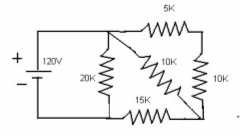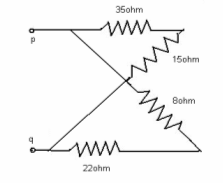# Network Theory - Electronics Engineering test questions

## Network Theory - Electronics Engineering test questions

(1) Non-Linear V-I characteristics is present in

(A) Transistor
(B) Filament bulb
(C) Vacuum diode
(D) All of the above

(2) An ideal current source has zero

(A) Internal resistance
(B) Internal conductance
(C) Ripple

(3) Kirchhoff’s law is applicable to

(A) AC and DC circuits
(B) AC circuits only
(C) DC circuits only
(D) Passive networks only

(4) Consider the system H (S) = S / S² + 2S + 100. Resonance frequency in rad/sec and bandwidth in rad/sec of the system are

(A) 10 and 5
(B) 10 and 10
(C) 10 and 2
(D) 10 and 1

(5) Valid circuit elements for a capacitor are

(A) Passive and bilateral
(B) Active and passive
(C) Passive and unilateral
(D) Active and bilateral

(6) To carry a current of 0.3 amperes, a 100 ohm resistor is needed in an electric circuit. A resistor you would select is

(A) 100 ohm, 7.5 watts
(B) 100 ohm, 10 watts
(C) 100 ohm, 1 watt
(D) 100 ohm, 5 watts

(7) One or more than one source of emf is present in

(A) Non-linear network
(B) Electric network
(C) Active network
(D) Passive network

(8) The current through an inductance follows

(A) A linear decay
(B) A linear growth
(C) An exponential growth
(D) An exponential decay

(9) For a 2 port network, the condition AD-BC = 1 implies that the network is

(A) Unilateral element network
(B) Lumped element network
(C) Lossless
(D) Reciprocal

(10) Let the inductance of the circuit is 16 H and a current of 6 ampere flows through it. The energy stored will be

(A) 288 joules
(B) 500 joules
(C) 144 joules
(D) 1563 joules

(11) In the iron core of an inductor an air gap is provided that prevents

(A) Hysteresis loss
(B) Core saturation
(C) Heat generation
(D) Flux leakage

(12) A coil has 200 turns. When the magnetic flux changes from 0.001 Wb to 0.0025 Wb in 0.1 sec, the emf in volts will be

(A) 4.5 V
(B) 1.5 V
(C) 3.0 V
(D) 7.5 V

(13) With zero initial condition at t = 0+, _________ acts as an open circuit

(A) Resistor
(B) Inductor
(C) Capacitor
(D) All of the above

(14) The coil inductance can be increased by

(A) Using core material of high relative permeability
(B) Reducing number of turns
(C) Increasing core length
(D) The inductance of a coil cannot be increased

ANSWER: Using core material of high relative permeability

For Questions 15 and 16 refer to the data given below:

Three resistances of values 1Ω, 2Ω and 3Ω are delta connected. Maintaining the same terminal conditions these three resistances are to be replaced by star connection

(15) The value of highest resistance in star will be

(A) 1/4 Ω
(B) 1/3 Ω
(C) 1 Ω
(D) 1/2 Ω

(16) The value of least resistance in star will be

(A) 1/4 Ω
(B) 1/3 Ω
(C) 1 Ω
(D) 1/2 Ω

#### Discussion

• RE: Network Theory - Electronics Engineering test questions -diksha p.chavhan (01/14/15)
• new indora ripablican nagar jaripatka nagpu
• Network Theory - Electronics Engineering test questions -Slesha Shirkey (11/12/13)
• For Questions 17 to 19 refer to the data given below:

DC supply of 200 V is applied to a series RL network where R = 20 Ω and L = 0.5 H

(17) At the instant of switching, the rate of change of current will be

(A) 400 A/sec
(B) 300 A/sec
(C) 200 A/sec
(D) 100 A/sec

(18) At t = L/R, the rate of change of current will be nearly

(A) 450 A/sec
(B) 350 A/sec
(C) 250 A/sec
(D) 150 A/sec

(19) The steady value of current will be

(A) 1 A
(B) 10 A
(C) 100 A
(D) 20 A

(20) Let R and L are in parallel connection. ω = 1000, Φ = +45° and |Z| = 50√2.
The value of R and L will be

(A) R =1Ω, L = 10H
(B) R =10Ω, L = 1H
(C) R =100Ω, L = 0.1H
(D) R =1000Ω, L = 0.01H

ANSWER: R =100Ω, L = 0.1H

For Questions 21 to 23 refer to the figure given below(21) The value of current supplied by the battery is

(A) 11.7 A
(B) 11.7 mA
(C) 1.17 A
(D) 1.17 mA

(22) The voltage developed across 20 K resistor will be

(A) 140 V
(B) 115 V
(C) 120 V
(D) 100 V

(23) The current through the cross branch 10 K resistor will be

(A) 34 mA
(B) 3.4 mA
(C) 340 mA
(D) 40 mA

For Questions 24 to 26 refer to the data given below:

Inherent resistance of an inductor is 50O and a time constant of 1.25 seconds. The inductor is now rewound with the same weight of wire of half the radius.

(24) Inductance value will be

(A) 1000 H
(B) 100 H
(C) 10 H
(D) 1 H

(25) The new constant will be

(A) More than 1.25 sec
(B) Less than 1.25 sec
(C) 1.25 sec
(D) Information provided is not sufficient

(26) When the inductor is connected to 600 V dc source, the stored energy will be nearly

(A) 200 J
(B) 281 J
(C) 140 J
(D) 100 J

(27) Two inductors when connected in parallel, the equivalent inductance is 4 H. When they are connected in series, the equivalent inductance is 20 H. The value of individual inductance should be

(A) 10 H and 10 H
(B) 8 H and 12 H
(C) 6.5 H and 12 H
(D) 4 H and 16 H

ANSWER: 4 H and 16 H

(28) What will be the voltage needed across pq so that the voltage drop across the 15 ohm resistor is 45 volts.(A) 150 V
(B) 200 V
(C) 100 V
(D) 50 V

(29) The open circuit I-V characteristic is

(A) A horizontal line through the origin
(B) A horizontal line above the origin
(C) A vertical line away from origin
(D) A vertical line through origin

ANSWER: A vertical line through origin

(30) The condition of a linear resistor, 0 < R < infinity represents

(A) Voltage controlled resistor
(B) Current controlled resistor
(C) Neither voltage controlled nor current controlled resistor
(D) Both voltage controlled and current controlled resistor

ANSWER: Both voltage controlled and current controlled resistor

(31) A non-linear resistor is represented as

(A) V + 10 i = 0
(B) 3V + i = 10
(C) V = i²
(D) All of the above

(32) The super-position theorem is applicable to

(A) Linear and non linear responses only
(B) Linear responses only
(C) Linear, non linear and time variant responses
(D) None of the above

(33) When one resistor of a delta-connected circuit is open, power will be

(A) Reduced by 1/3
(B) Zero
(C) Reduced to 1/3
(D) Unaltered

(34) In 3 phase star connected network, line voltage is same as

(A) 3 phase voltage
(B) √3phase voltage
(C) 1/√3 phase voltage
(D) Phase voltage

(35) Consider the Norton equivalent circuit. When a 1 ohm resistor is connected across the input terminals, equivalent resistor, equivalent voltage and current will be

(A) 2/3 ohm, -10 V, -6 A
(B) 2/3 ohm, 10 V, -6 A
(C) 2/3 ohm, -5 V, 6 A
(D) 2/3 ohm, 5 V, 6 A

ANSWER: 2/3 ohm, 10 V, -6 A

(36) Millman’s theorem yields

(A) Equivalent impedance
(B) Equivalent resistance
(C) Equivalent voltage source
(D) Equivalent voltage or current source

ANSWER: Equivalent voltage or current source

For Questions 37 to 39 refer to the figure shown below:(37) The impedance Za of the star connection will be

(A) (1 + j 5.1) Ω
(B) (0.3 + j 7.4) Ω
(C) (1.5 + j 8.1) Ω
(D) (2 + j 10.5) Ω

ANSWER: (0.3 + j 7.4) Ω

(38) The impedance Zb of the star connection will be

(A) (13 + j 13.5) Ω
(B) (0.3 + j 7.4) Ω
(C) (1 + j 7) Ω
(D) (18 + j 3.5) Ω

ANSWER: (13 + j 13.5) Ω

(39) The impedance Zc of the star connection will be

(A) (8 + j 12) Ω
(B) (1 + j 1.8) Ω
(C) (24 + j 13.8) Ω
(D) (8 + j 1.8) Ω

ANSWER: (8 + j 1.8) Ω

(40) To solve a network, the number of independent equations is equal to

(A) The number of chords
(B) The number of branches
(C) Sum of the number of chords and branches
(D) Sum of the number of chords, branches and nodes